# Jaeger composition product

(diff) ← Older revision | Latest revision (diff) | Newer revision → (diff)

A formula for the Jones–Conway polynomial, describing it as a sum of products of the Jones–Conway polynomials of pieces of the diagram. It has its root in the statistical mechanics model of the Jones–Conway polynomial by V.F.R. Jones. It has been applied to periodic links and to the building of a Hopf algebra structure on the Jones–Conway skein module of the product of a surface and an interval [a3], [a4], [a2].

To define the Jaeger composition product it is convenient to work with the following regular isotopy variant of the Jones–Conway polynomial: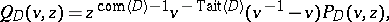where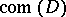is the number of link components andis the algebraic sum of the signs of the crossings of. It is also convenient to add the empty link,, to the set of links and put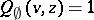.satisfies the skein relationand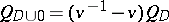. The advantage of working with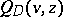is that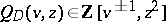(no negative powers of) and that the Jaeger composition product has a nice simple form. Indeed ([a1]): Letbe a diagram of an oriented link in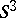, thenThe meaning of the used symbols is as follows. To define, consideras a-valent graph. Let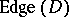denote the set of edges of the graph. A-labelling ofis a function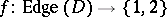such that around a vertex the following labellings are allowed:Figure: j130010a

The set of-labellings ofis denoted by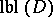. The edges ofwith labelform an oriented link diagram, denoted by. The vertices ofwhich are neither in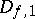nor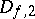are called-smoothing vertices of. Let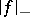(respectively,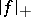) denote the number of negative (respectively, positive)-smoothing vertices of. Letand define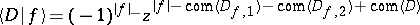. Finally,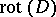denotes the rotational number of, i.e. the sum of the signs of the Seifert circles of, where the sign of such a circle isif it is oriented counterclockwise and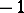otherwise.

How to Cite This Entry:
Jaeger composition product. Encyclopedia of Mathematics. URL: http://encyclopediaofmath.org/index.php?title=Jaeger_composition_product&oldid=18470
This article was adapted from an original article by Jozef Przytycki (originator), which appeared in Encyclopedia of Mathematics - ISBN 1402006098. See original article